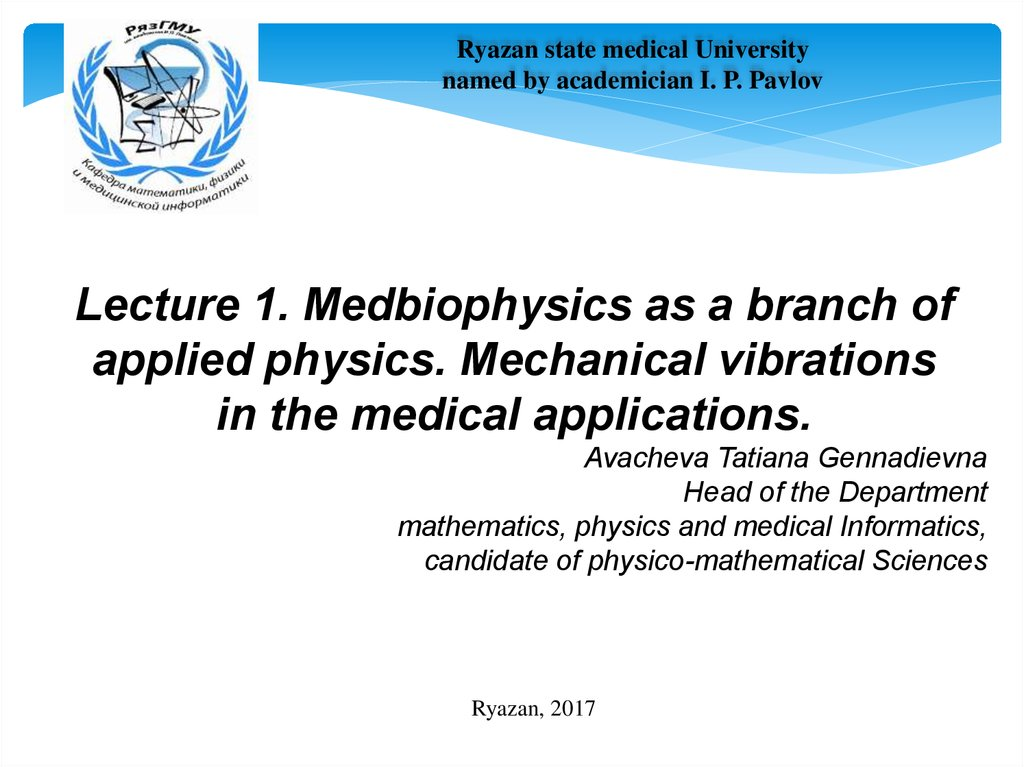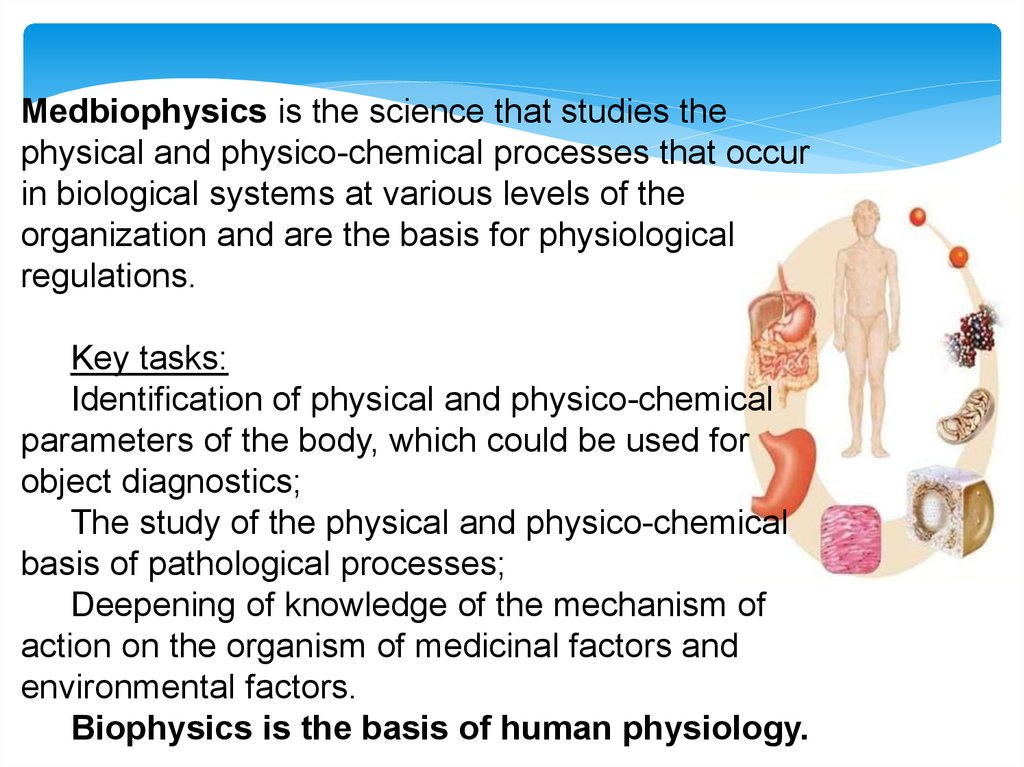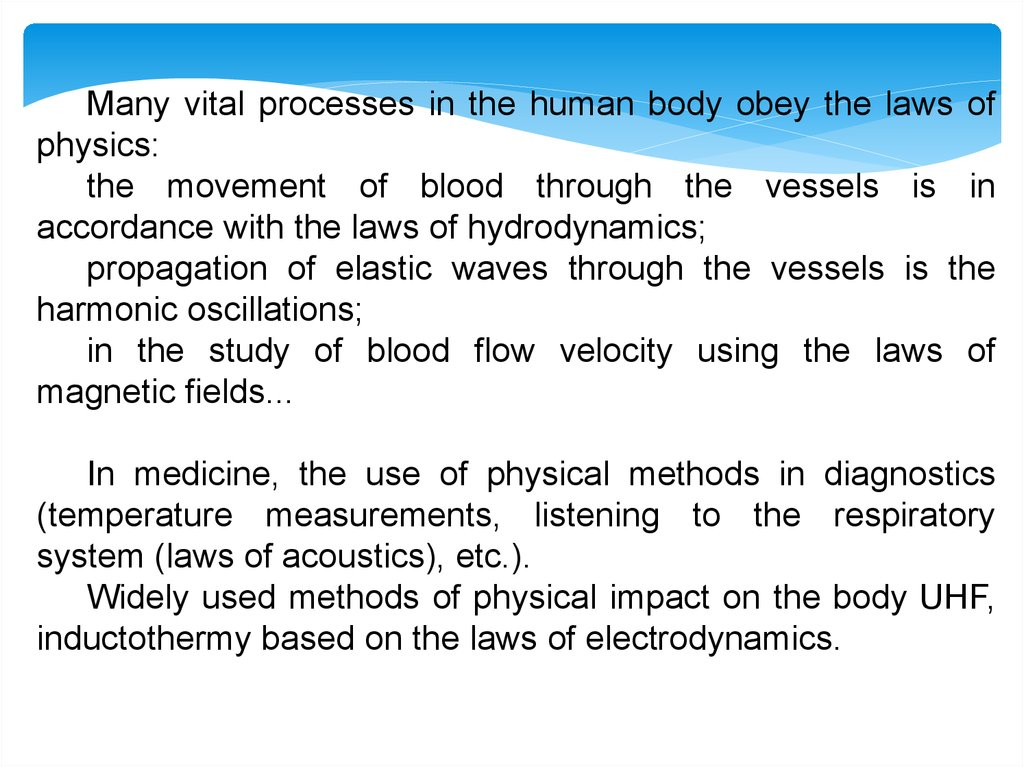# Medbiophysics as a branch of applied physics. Mechanical vibrations in the medical applications

## 1.

Ryazan state medical University
named by academician I. P. Pavlov
Lecture 1. Medbiophysics as a branch of
applied physics. Mechanical vibrations
in the medical applications.
mathematics, physics and medical Informatics,
candidate of physico-mathematical Sciences
Ryazan, 2017

## 2.

Medbiophysics is the science that studies the
physical and physico-chemical processes that occur
in biological systems at various levels of the
organization and are the basis for physiological
regulations.
Identification of physical and physico-chemical
parameters of the body, which could be used for
object diagnostics;
The study of the physical and physico-chemical
basis of pathological processes;
Deepening of knowledge of the mechanism of
action on the organism of medicinal factors and
environmental factors.
Biophysics is the basis of human physiology.

## 3.

Many vital processes in the human body obey the laws of
physics:
the movement of blood through the vessels is in
accordance with the laws of hydrodynamics;
propagation of elastic waves through the vessels is the
harmonic oscillations;
in the study of blood flow velocity using the laws of
magnetic fields...
In medicine, the use of physical methods in diagnostics
(temperature measurements, listening to the respiratory
system (laws of acoustics), etc.).
Widely used methods of physical impact on the body UHF,
inductothermy based on the laws of electrodynamics.

## 4.

•Periodic mechanical
processes in the living body
«Each person is a complex
oscillatory system. "
N. Wiener
Oscillations are processes that repeat in time.
In this case, the system repeatedly deviates from its equilibrium state and
returns to it again each time.
Depending on the physical nature of the repeating process, oscillations are
distinguished: mechanical, electrical, etc.
In this lecture, mechanical vibrations are considered.
Repeating processes continuously occur inside any living organism.

## 5.

For example:
•Дыхательные движения грудной клетки;
•Rhythmic contractions of the heart;
•Blood flow to the arteries (pulse);
•Breathing movements of the chest;
•we hear and talk due to fluctuations in the
eardrums and vocal cords;
•when walking our feet make oscillatory
movements.
•The atoms from which we are forming
fluctuate.

## 6.

Examples of oscillatory
systems

## 7.

Among the various modes of vibration, the most simple form is harmonic oscillation, i.e. such
that the oscillating quantity varies as a function of time according to the law of the sine or
cosine.
Their significance is due to the following reasons. First, fluctuations in nature and in
technology often have a character very close to the harmonic, and, secondly, periodic
processes of a different form (with a different time dependence) can be represented as the
imposition of several harmonic oscillations.
x
xm
0
xm
π/2
π
3π/2

T/4
T/2
3T/4
T
x = Xm sin(ω t + φ0)
x = Xm cos(ω t + φ0)
φ
t
harmonic oscillation
equation

## 8.

MAIN CHARACTERISTICS OF
VIBRATIONAL MOVEMENT
x = Xm sin(ω t + φ0)
• x – the displacement of the point from the
equilibrium position at a given time (instantaneous
value).
• А, Xm – the module of the maximum
displacement of a point from an equilibrium position
is called the amplitude;
•φ
= ωt + φ0
– phase of oscillation, which
determines the state of the oscillatory system at any
•φ0 – initial phase of oscillation

## 9.

MAIN CHARACTERISTICS OF
VIBRATIONAL MOVEMENT
x = Xm sin(ω t + φ0)
• Т –the time of one complete oscillation is called the
period
Т = t/n,
where n is the number of complete oscillations in time t
• - the number of oscillations per unit time is called the
frequency
= 1/Т – linear frequency
= n/t
ω = 2π – cyclic oscillation frequency
ω = 2π
[ 1/s=Hz]
= 2π/Т period and frequency relationship

## 10.

Graphs of the dependence of displacement on time for
х(0) = А и х(0) = 0

## 11.

The harmonic oscillation graph is a sinusoidal or cosine wave.
In all three cases for blue curves φ0 = 0:
The red curve differs from the blue only by the larger amplitude(x'm > xm);
The red curve differs from the blue only by the value of the period (T' = T / 2);
The red curve differs from blue only in the value of the initial phase (rad).

## 12.

All fluctuations are divided into 3 groups:
1. Free undamped,
2. free decaying,
3. forced.
Free vibrations are called such vibrations that occur in a system left to itself
after it has been taken out of equilibrium.
Example: oscillations of a ball suspended on a string. In order to cause fluctuations, you
either need to push the ball, or, taking it aside, let it go. When the ball is shaken, the
kinetic energy is reported, and in the case of a deviation, the potential energy is
reported.
Mathematical pendulum
E п о т m gh
T 2
Free oscillations are made due to the initial energy reserve.
E кин
l
g
2
m
2

## 13.

Free undamped oscillations
Free oscillations can be undamped only in the absence of frictional force.
Otherwise, the initial energy reserve will be spent on overcoming it, and the range of
fluctuations will decrease.
As an example, consider the vibrations of a body suspended on a weightless spring, arising
after the body was turned down, and then released.
m
T 2
k
E кин
E пот
m 2
2
kx
2
2

## 14.

From the side of the stretched spring to the body acts the elastic
force F, proportional to the magnitude of the displacement х:
k - spring rigidity and depends on its dimensions
and material.
The sign "-" indicates that the force of elasticity is
always directed in the direction opposite to the
direction of displacement, to the equilibrium
position.
In the absence of friction, the elastic force F is the
only force acting on the body. According to
Newton's second law (ma = F):
kx mx' '
x' '
- second derivative by time

## 15.

After transferring all the terms to the left
and dividing by the mass of the body (m),
we obtain the differential equation of free
oscillations in the absence of friction:
The solution of this equation is the harmonic function
The value of ω0 (1.6) turned out to be equal to the cyclic
frequency. This frequency is called own.
Thus, free oscillations in the absence of friction are harmonic.

## 16.

Speed and acceleration of the oscillating body:
change according to the same law with a phase shift

## 17.

Energy of harmonic oscillations.
The energy of the oscillating system consists of potential and kinetic energies.
E Екин Епот
Екин
m 2
2
Епот
kx
2
2
m 2 kx2
Е
2
2
x A cos( 0t 0 )
A 0 sin( 0t 0 )
02
m 2 2
k 2
2
Е A 0 sin ( 0t 0 ) A cos 2 ( 0t 0 )
2
2
k
m
m 02 k
2
kA2
kA
Е
sin 2 ( 0t 0 ) cos 2 ( 0t 0 )
2
2
kA2
Е
2
- the total energy remains constant = const

## 18.

Damped oscillations
In real conditions, any oscillatory system is under the influence of frictional forces
(resistance). At the same time, part of the mechanical energy is converted into internal
energy of the thermal motion of atoms and molecules, and the oscillations become
damped.
Damped are called oscillations whose amplitude decreases with time.

## 19.

Differential equation of damped oscillations
x' ' 2 x' 02 x 0
where β is the attenuation coefficient.
The solution of this equation is:
x A0 e t cos( t 0 )
The ratio of two amplitudes separated from each other by a period T,
are called the damping decrement:
At
D
e Т
At Т
ln D T
- logarithmic decrement decrement.
02 2 2
- frequency of damped oscillations
A A0e t

## 20.

Forced oscillations. Resonance
In order for the oscillations not to decay, it is necessary
to inform the system of additional energy, i.e. to act on
the oscillating system by periodic force. Such oscillations
are called forced. Forced oscillations are made with a
frequency equal to the frequency of the change in the
external force.

## 21.

The amplitude of forced mechanical oscillations reaches its
maximum value in the case when the frequency of the
driving force coincides with the frequency of the oscillatory
system.
This phenomenon is called resonance.
For example, if you periodically pull the cord in time with its
own oscillations, we notice an increase in the amplitude of
its oscillations.

## 22.

The phenomenon of resonance can be the cause of the
destruction of machines, buildings, bridges, if their own
frequencies coincide with the frequency of the periodically
acting force.
So in the USA, a strong wind, whose frequency coincided with the frequency of
oscillations of the bridge, led to its destruction.
Let's look at the video.

## 23.

Positive resonance value
The resonance
phenomenon is used in
devices.
Frequency meter - a
device for measuring the
frequency of oscillations

## 24.

Positive resonance value
Wind musical instruments use this phenomenon

## 25.

Resonance cavities
of the vocal
apparatus
The hearing is also
based on resonance

## 27.

Human body vibrations and their registration
The analysis of oscillations created by the human body or its individual parts is widely used in
medical practice.
Walking is a complex, periodic locomotor process that occurs as a result of the coordinated
activity of the skeletal muscles of the trunk and extremities. The analysis of the walking
process gives many diagnostic signs.
Рис. 1.8. Вертикальное смещение ЦМ тела человека во время ходьбы

## 28.

Mechanical oscillations of the heart
There are various methods of heart research, which are based on mechanical
periodic processes.
Ballistocardiography (BCG) is a method for studying the mechanical
manifestations of cardiac activity, based on the recording of pulsed microscopic
movements of the body, caused by the ejection of blood from the ventricles of
the heart into large vessels.
Apekskardiography (AKG) - a method of graphical recording of low-frequency
vibrations of the thorax in the region of apical stimulation caused by the work of
the heart. Registration of an apekskardiogram is usually done on a multichannel
electrocardiograph using a piezocrystalline sensor, which is a converter of
mechanical vibrations into electrical oscillations.

## 29. Positive resonance value Wind musical instruments use this phenomenon

Vibration
The work of many mechanisms is associated with the occurrence of
vibrations, which are transmitted to a person and have a harmful effect on
him.
Vibration - forced oscillations of the body, under which either the whole body
vibrates as a single whole, or its individual parts oscillate with different
amplitudes and frequencies.
Long-term exposure to vibrations causes persistent disturbances in normal
physiological functions in the body.
Oscillations with a frequency of 3-5 Hz cause the reactions of the vestibular
apparatus, vascular disorders. At frequencies of 3-15 Hz, there are disorders
associated with resonance oscillations of individual organs (liver, stomach,
head) and the body as a whole. Oscillations with frequencies of 11-45 Hz
cause visual impairment, nausea, vomiting. At frequencies exceeding 45 Hz,
cerebral vessels are damaged, blood circulation is disturbed, etc.
At a frequency of 10,000 Hz there is heating of tissues, destruction of cells.

## 30.

Vibration
At the same time, in a number of cases, vibrations are used in medicine.
Using high-frequency vibrating apparatuses allows drilling a hole in a tooth in a
complex shape.
Vibration is also used for massage. With manual massage, the massaged tissues
are brought into oscillatory motion with the help of the hands of a massage
therapist.